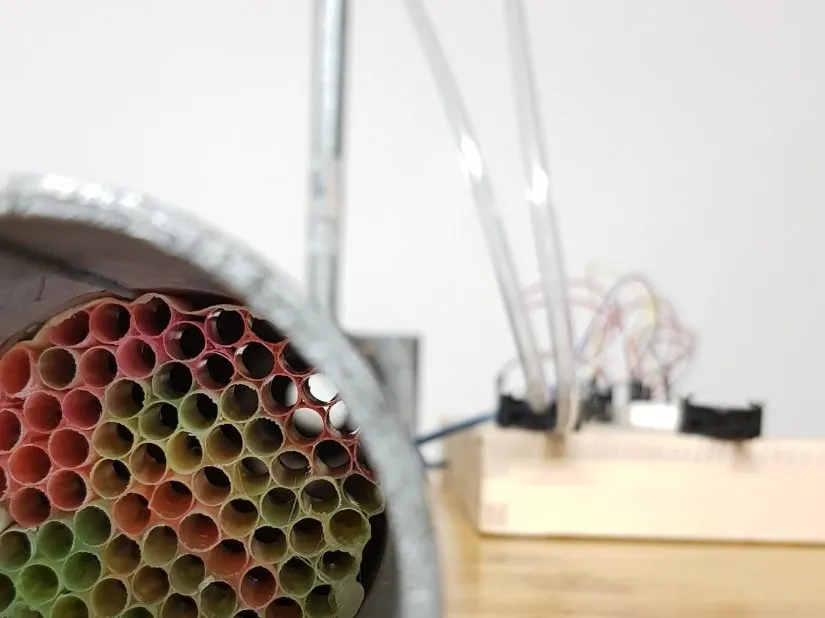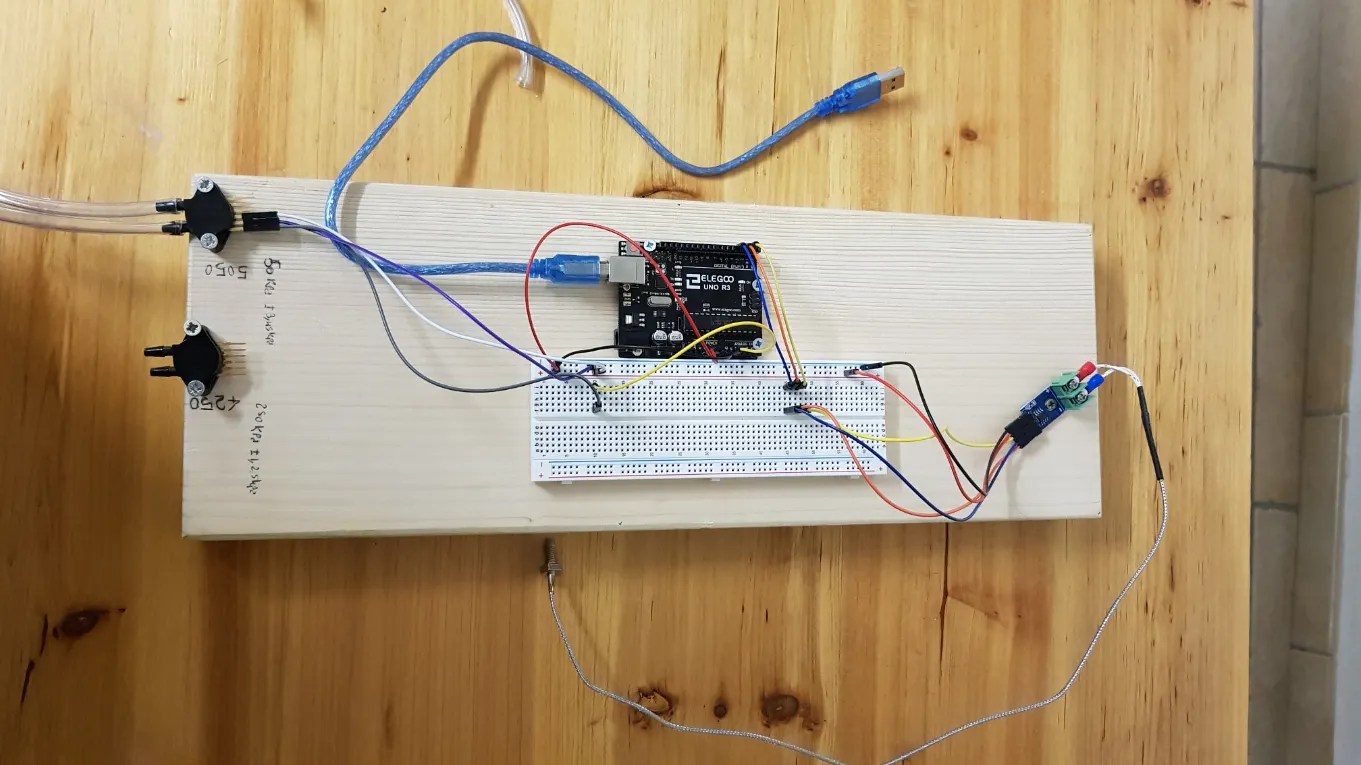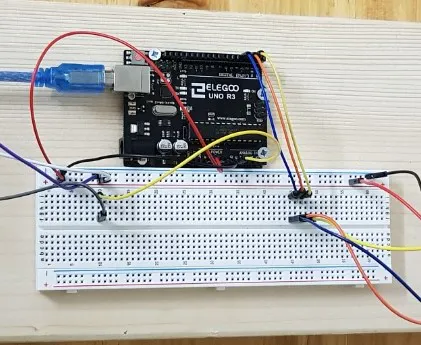# Orifice Plate and Fluid dynamics!

It's a device which allows you to compute the mass flow within a pipe. The implementation with arduino is very easy.## Things used in this project

### Hardware components

 NXP MPX5050dp
×1
 MAX6675
×1Arduino UNO
×1

### Software apps and online servicesArduino IDE
 MICROSOFT EXCEL

## Schematics

### connections### connections (2)## Code

### SENSOR_ORIFICE_PLATE.ino

C/C++
This code show you the pression and temperature values. Then with excel, you can compute the flow through the Numerical analysis.
```#include <MemoryFree.h>

#include "max6675.h"

//variables initialization
float Vs = 5.1;

float T_1=0;

// START Thermocouple
int thermo1DO = 3;
int thermo1CS = 4;
int thermo1CLK = 2;
MAX6675 ktc1(thermo1CLK, thermo1CS, thermo1DO);

// START MPX5050
int PIN_P1 = A0;
float Analog_P1 = 0;
float Vout_P1 = 0;
int P_1 = 0;

//SETUP

void setup() {
Serial.begin(9600);      // START serial communication

}

// LOOP
void loop() {

Vout_P1 = ((Analog_P1 * 0.00369) + 0.04);
P_1 = (Vs * Vout_P1);
delay(1000);

// print data
Serial.print("T=");
Serial.print(T_1);
Serial.print("C");
Serial.print("\n");
Serial.print("P = ");
Serial.print(P_1,1);
Serial.print("Kpa");
Serial.print("\n");
}
```

## Credits

### cicchine_ing

0 projects • 1 follower
I'm a mechanical engineer, graduated in Modena (Unimore).